# Area chart in ggplot2 with geom_area

## Sample data set

Consider the following data frame that contains the price of three european stock indices.

``df <- as.data.frame(EuStockMarkets[, 1:3])``

## Area chart of a single line

In order to create an area chart with a column of the input data frame you can pass the dates (if available) or an index to `x` and the variable of interest to `y` and then use `geom_area`.

``````# install.packages("ggplot2")
library(ggplot2)

# Area chart
ggplot(df, aes(x = seq_along(SMI), y = SMI)) +
geom_area()``````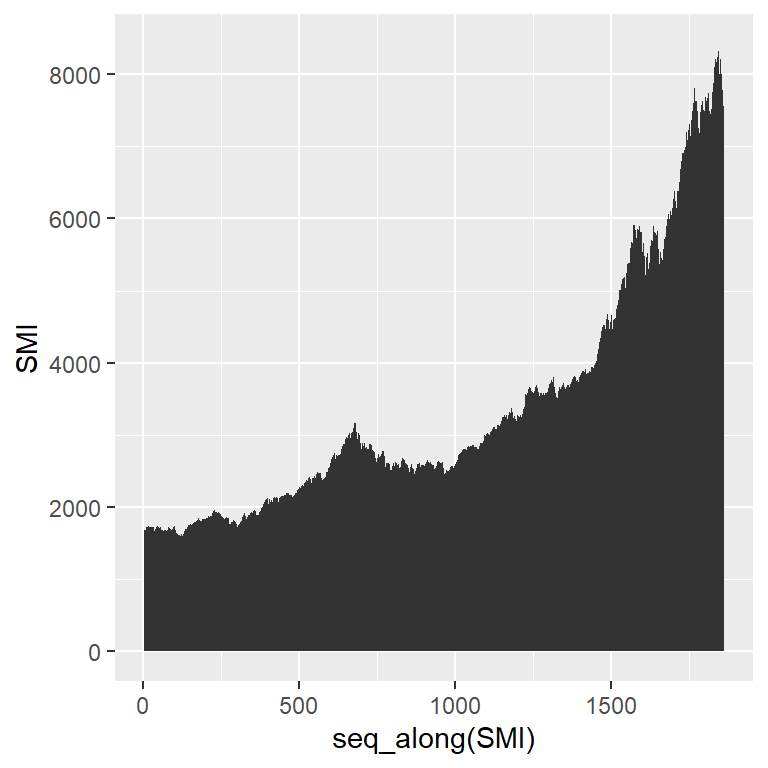Fill the area

By default the area will be filled with a very dark gray color. However, you can change it passing a color to the `fill` argument.

``````# install.packages("ggplot2")
library(ggplot2)

# Area chart
ggplot(df, aes(x = seq_along(SMI), y = SMI)) +
geom_area(fill = 4)``````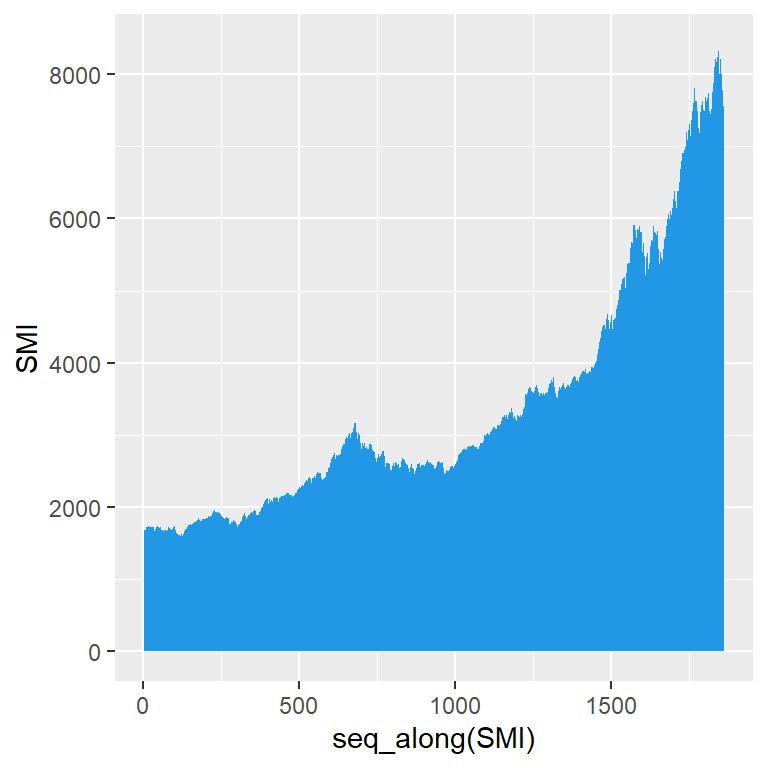Transparency of the area

You can also change the transparency of the area with the `alpha` argument of the function.

``````# install.packages("ggplot2")
library(ggplot2)

# Area chart
ggplot(df, aes(x = seq_along(SMI), y = SMI)) +
geom_area(fill = 4,
alpha = 0.5)``````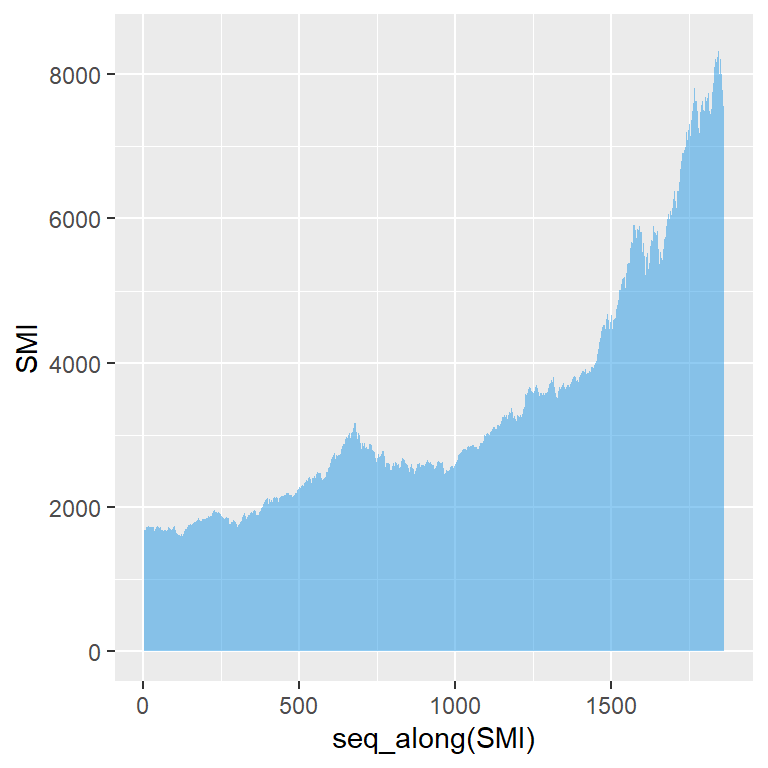Line customization

The upper line of the area can be customized with several arguments, such as `color`, `lwd` or `linetype`.

``````# install.packages("ggplot2")
library(ggplot2)

# Area chart
ggplot(df, aes(x = seq_along(SMI), y = SMI)) +
geom_area(fill = 4,
alpha = 0.5,
color = 1,    # Line color
lwd = 0.5,    # Line width
linetype = 1) # Line type``````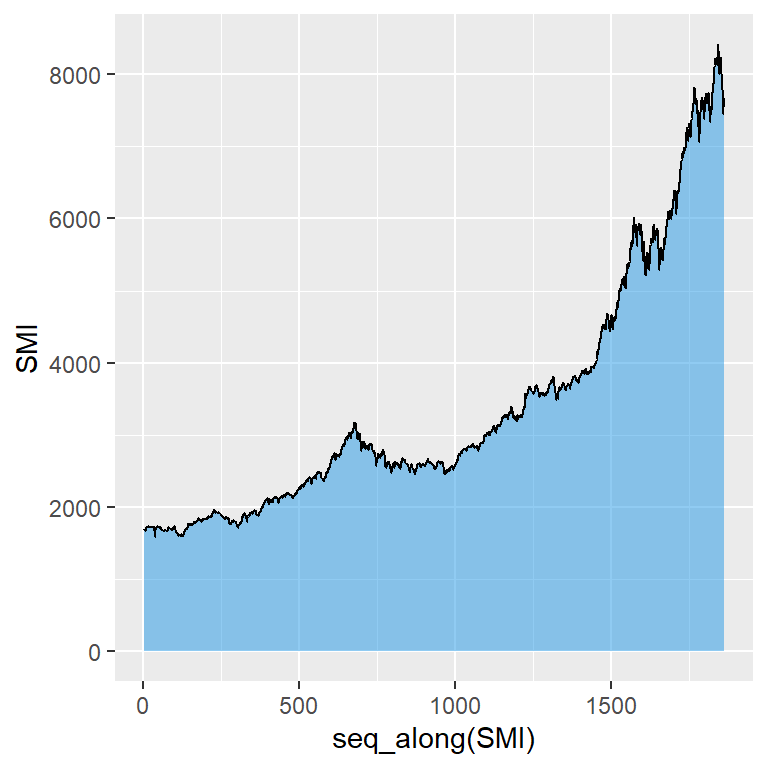## Area chart of several lines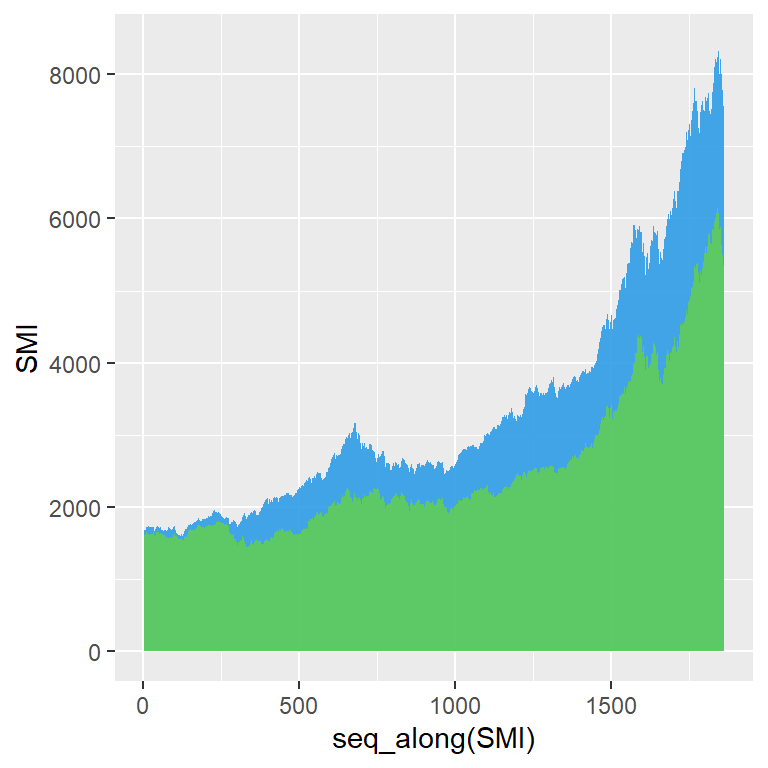In case you want to add more variables you will need to specify the aesthetics inside each `geom_area` for each variable. Note that if the number of areas to add is more than two or three you should consider creating a stacked area chart.

``````# install.packages("ggplot2")
library(ggplot2)

# Area chart
ggplot(df) +
geom_area(aes(x = seq_along(SMI), y = SMI),
fill = 4, alpha = 0.85) +
geom_area(aes(x = seq_along(DAX), y = DAX),
fill = 3, alpha = 0.85)``````